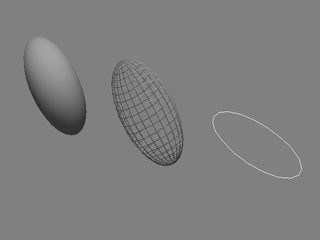# class mrpt::opengl::CEllipsoid2D

A 2D ellipse on the XY plane, centered at the origin of this object pose.

The color is determined by the RGBA fields in the class “CRenderizable”. Note that a transparent ellipse can be drawn for “0<alpha<1” values. If any of the eigen value of the covariance matrix of the ellipsoid is zero, it will not be rendered.

Please read the documentation of CGeneralizedEllipsoidTemplate::setQuantiles() for learning the mathematical details about setting the desired confidence interval.opengl::Scene

```#include <mrpt/opengl/CEllipsoid2D.h>

class CEllipsoid2D: public mrpt::opengl::CGeneralizedEllipsoidTemplate
{
public:
// typedefs

typedef mrpt::math::CMatrixFixed<double, DIM, DIM> cov_matrix_t;
typedef mrpt::math::CMatrixFixed<double, DIM, 1> mean_vector_t;

// construction

CEllipsoid2D();

//
methods

virtual void render(const RenderContext& rc) const;
virtual void freeOpenGLResources();
void renderUpdateBuffers() const;
virtual void onUpdateBuffers_Wireframe();
virtual void onUpdateBuffers_Triangles();
void set2DsegmentsCount(unsigned int N);
virtual bool traceRay(const mrpt::poses::CPose3D& o, double& dist) const;
void setCovMatrixAndMean(const MATRIX& new_cov, const VECTOR& new_mean);
cov_matrix_t getCovMatrix() const;
void setCovMatrix(const MATRIX& new_cov);
void setQuantiles(float q);
float getQuantiles() const;
void setNumberOfSegments(const uint32_t numSegments);
void enableDrawSolid3D(bool v);
virtual bool traceRay(] const mrpt::poses::CPose3D& o, ] double& dist) const;
virtual const mrpt::rtti::TRuntimeClassId* GetRuntimeClass() const;
};```

## Inherited Members

```public:
// structs

struct OutdatedState;
struct RenderContext;
struct State;

//
methods

virtual void render(const RenderContext& rc) const = 0;
virtual void renderUpdateBuffers() const = 0;
virtual void freeOpenGLResources() = 0;
virtual void onUpdateBuffers_Triangles() = 0;
virtual void onUpdateBuffers_Wireframe() = 0;```

## Typedefs

`typedef mrpt::math::CMatrixFixed<double, DIM, DIM> cov_matrix_t`

The type of fixed-size covariance matrices for this representation.

`typedef mrpt::math::CMatrixFixed<double, DIM, 1> mean_vector_t`

The type of fixed-size vector for this representation.

## Methods

`virtual void render(const RenderContext& rc) const`

Implements the rendering of 3D objects in each class derived from CRenderizable.

This can be called more than once (one per required shader program) if the object registered several shaders.

renderUpdateBuffers

`virtual void freeOpenGLResources()`

Free opengl buffers.

`void renderUpdateBuffers() const`

Called whenever m_outdatedBuffers is true: used to re-generate OpenGL vertex buffers, etc.

before they are sent for rendering in render()

`virtual shader_list_t requiredShaders() const`

Returns the ID of the OpenGL shader program required to render this class.

`virtual void onUpdateBuffers_Wireframe()`

Must be implemented in derived classes to update the geometric entities to be drawn in “m_*_buffer” fields.

`virtual void onUpdateBuffers_Triangles()`

Must be implemented in derived classes to update the geometric entities to be drawn in “m_*_buffer” fields.

`void set2DsegmentsCount(unsigned int N)`

The number of segments of a 2D ellipse (default=20)

`virtual bool traceRay(const mrpt::poses::CPose3D& o, double& dist) const`

Ray tracing.

`void setCovMatrixAndMean(const MATRIX& new_cov, const VECTOR& new_mean)`

Set the NxN covariance matrix that will determine the aspect of the ellipsoid - Notice that the covariance determines the uncertainty in the parameter space, which would be transformed by derived function.

`cov_matrix_t getCovMatrix() const`

Gets the current uncertainty covariance of parameter space.

`void setCovMatrix(const MATRIX& new_cov)`

Like setCovMatrixAndMean(), for mean=zero.

`void setQuantiles(float q)`

Changes the scale of the “sigmas” for drawing the ellipse/ellipsoid (default=3, ~97 or ~98% CI); the exact mathematical meaning is: This value of “quantiles” q should be set to the square root of the chi-squared inverse cdf corresponding to the desired confidence interval.

Note that this value depends on the dimensionality. Refer to the MATLAB functions chi2inv() and chi2cdf().

Some common values follow here for the convenience of users:

• Dimensionality=3 (3D ellipsoids):

• 19.8748% CI -> q=1

• 73.8536% CI -> q=2

• 97.0709% CI -> q=3

• 99.8866% CI -> q=4

• Dimensionality=2 (2D ellipses):

• 39.347% CI -> q=1

• 86.466% CI -> q=2

• 98.8891% CI -> q=3

• 99.9664% CI -> q=4

• Dimensionality=1 (Not aplicable to this class but provided for reference):

• 68.27% CI -> q=1

• 95.45% CI -> q=2

• 99.73% CI -> q=3

• 99.9937% CI -> q=4

`float getQuantiles() const`

Refer to documentation of setQuantiles()

`void setNumberOfSegments(const uint32_t numSegments)`

Set the number of segments of the surface/curve (higher means with greater resolution)

`void enableDrawSolid3D(bool v)`

If set to “true”, a whole ellipsoid surface will be drawn, or if set to “false” (default) it will be drawn as a “wireframe”.

`virtual bool traceRay(] const mrpt::poses::CPose3D& o, ] double& dist) const`

Ray tracing

`virtual const mrpt::rtti::TRuntimeClassId* GetRuntimeClass() const`

Returns information about the class of an object in runtime.